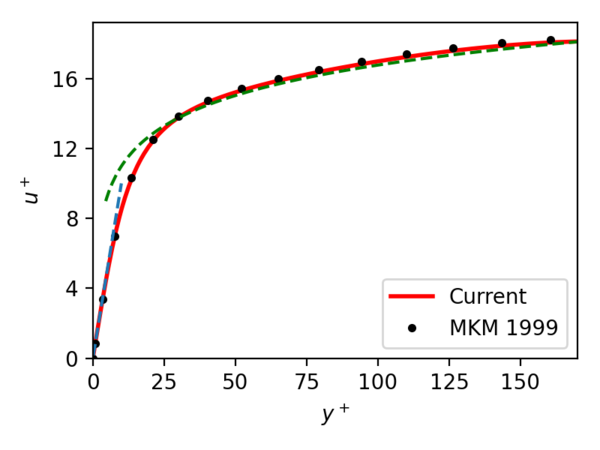# DNS 1-2 Computational Details

(diff) ← Older revision | Latest revision (diff) | Newer revision → (diff)

# Computational Details

## Computational approach

The computations are performed using PyFR version 1.12.0, a python based framework for solving advection-diffusion type problems using the Flux Reconstruction approach of Huynh. The details of the numerical approach are available here.

## Spatial and temporal resolution, grids

The domain is discretised into $62\times 29\times 60$hexahedral elements. For a polynomial order of 5, this yields 174 solution points in the transverse direction and about $23\times 10^{6}$in total. The grid is uniform in the spanwise and streamwise directions, but clustered near the walls in transverse direction. Iyer et al. (2019) observed that the grid resolution and polynomial order used in these calculations are sufficient enough to guarantee mesh independence of velocity statistics. The resolution is also sufficient to accurately capture the linear dependence of $u^{+}$on $y^{+}$and the log law as seen in the figure. There is also good agreement in the inner, transition and outer layers with the results of Moser et al.  (hereafter, MKM1999) that is shown in the same figure. The dashed lines represent the linear (blue) and log-law (green) variation of velocity with respect to $y^{+}$. The velocity profile in semi-log scale is given in figure 3.Figure 2: Averaged streamwise velocity profile in the turbulent channel compared with the results of Moser et al. (1999)

An explicit RK45 scheme is used to advance the solution in time. The order of accuracy of the solution changes as a function of time, as discussed in Witherden et al. (2014). The grid used for the simulations is available on the ERCOFTAC database.

## Computation of statistical quantities

PyFR computes volumetric time average quantities by accumulating averages every 50 time steps over a prescribed time period, which is 100 time units for the channel flow simulations. The average calculation is started after 3000 convective time unit in windowed mode. The outputs from the different averaging windows are then combined together. For the channel flow, PyFR accumulates the average fields $({\bar {u}},{\bar {p}},{\overline {\frac {du}{dx}}},\ldots )$, second-order correlations $({\overline {uv}},{\overline {up}},{\overline {u{\frac {dp}{dx}}}},\ldots )$and third-order correlations $({\overline {uuu}},\ldots )$in the aforementioned process. These statistics are then averaged in the streamwise and spanwise directions. From these statistical quantities, the individual terms in the turbulent stress budget equations are computed. Wall-normal gradients of the averaged quantities are calculated using a cubic spline interpolation.

1. F.D. Witherden, A.M. Farrington & P.E. Vincent, PyFR: An open source framework for solving advection–diffusion type problems on streaming architectures using the flux reconstruction approach, Computer Physics Communications, 185 (3028-2040), 2014
2. A. Iyer, F. D. Witherden, S. I. Chernyshenko & P. E. Vincent, Identifying eigenmodes of averaged small-amplitude perturbations to turbulent channel flow, Journal of Fluid Mechanics, 875 (758-780), 2019
3. R. D. Moser, J. Kim, J. & N. N. Mansour, Direct numerical simulation of turbulent channel flow up to $Re_{\tau }=590$, Physics of Fluids, 11 (4), 1999

Contributed by: Arun Soman Pillai, Lionel Agostini, Peter Vincent — Imperial College London